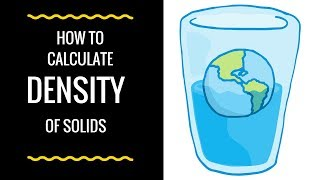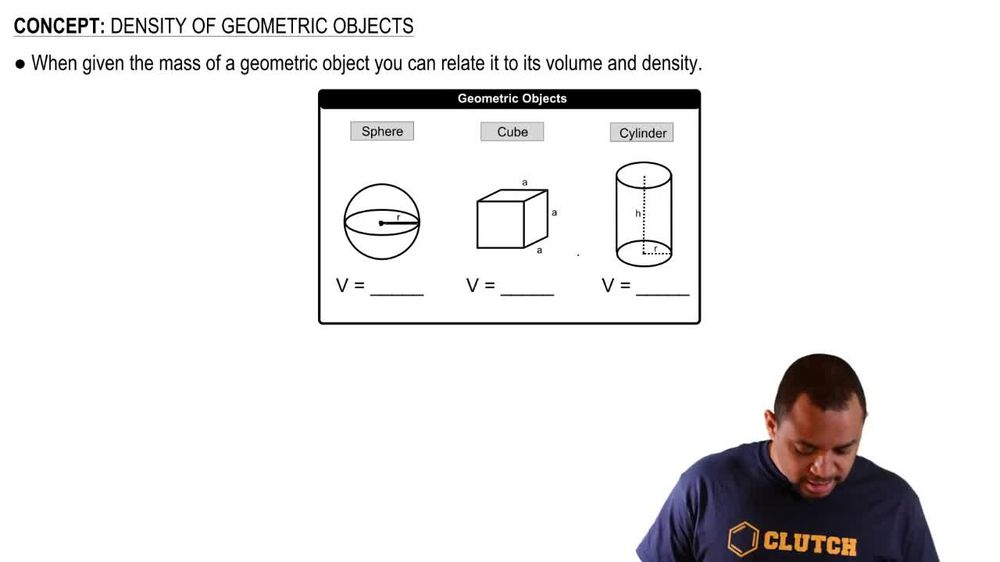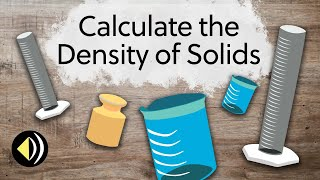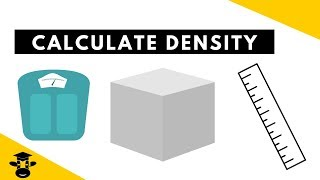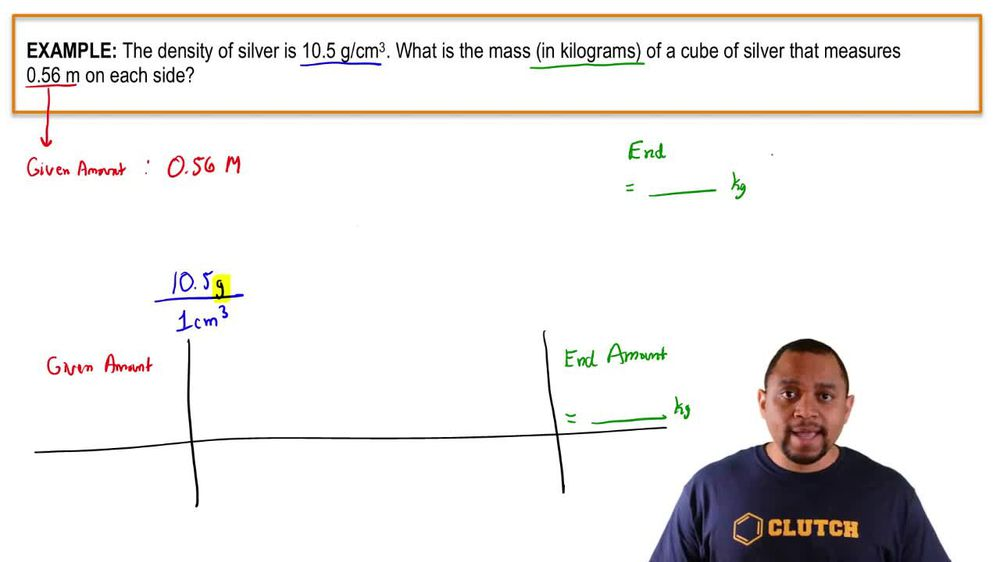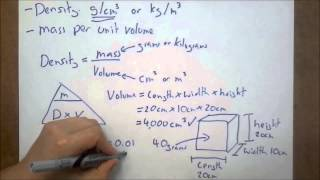Start typing, then use the up and down arrows to select an option from the list.
1. 1. Intro to General Chemistry2. Density of Geometric Objects
Problem

# A thief uses a can of sand to replace a solid gold cylinder that sits on a weight-sensitive, alarmed pedestal. The can of sand and the gold cylinder have exactly the same dimensions (length = 22 and radius = 3.8 cm). a. Calculate the mass of each cylinder (ignore the mass of the can itself). (density of gold = 19.3 g>cm3, density of sand = 3.00 g>cm3) b. Does the thief set off the alarm? Explain.

Relevant Solution4m
Play a video: